#### Singular complex

Simplicial complexes are useful in applications such as geometric modeling and computer graphics for computing the topology of models. Due to the complicated topological spaces, implicit, nonlinear models, and decomposition algorithms that arise in motion planning, they are insufficient for the most general problems. A singular complex is a generalization of the simplicial complex. Instead of being limited to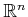, a singular complex can be defined on any manifold,(it can even be defined on any Hausdorff topological space). The main difference is that, for a simplicial complex, each simplex is a subset of; however, for a singular complex, each singular simplex is actually a homeomorphism from a (simplicial) simplex into a subset of.

To help understand the idea, first consider a 1D singular complex, which happens to be a topological graph (as introduced in Example 4.6). The interval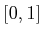is a-simplex, and a continuous path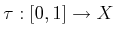is a singular-simplex because it is a homeomorphism ofto the image of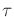in. Suppose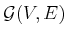is a topological graph. The cells are subsets ofthat are defined as follows. Each point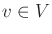is a 0-cell in. To follow the formalism, each is considered as the image of a function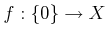, which makes it a singular 0-simplex, because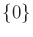is a 0-simplex. For each path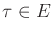, the corresponding-cell is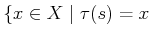for some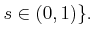(6.4)

Expressed differently, it is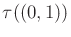, the image of the path, except that the endpoints are removed because they are already covered by the 0-cells (the cells must form a partition).

These principles will now be generalized to higher dimensions. Since all balls and simplexes of the same dimension are homeomorphic, balls can be used instead of a simplex in the definition of a singular simplex. Let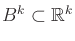denote a closed,-dimensional unit ball,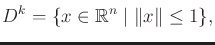(6.5)

in which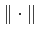is the Euclidean norm. A singular-simplex is a continuous mapping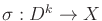. Let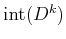refer to the interior of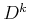. For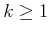, the-cell,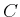, corresponding to a singular-simplex,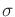, is the image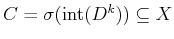. The 0-cells are obtained directly as the images of the 0 singular simplexes. Each singular 0-simplex maps to the 0-cell in. Ifis restricted to, then it actually defines a homeomorphism betweenand. Note that both of these are open sets if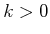.

A simplicial complex requires that the simplexes fit together nicely. The same concept is applied here, but topological concepts are used instead because they are more general. Let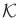be a set of singular simplexes of varying dimensions. Let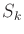denote the union of the images of all singular-simplexes for all.

A collection of singular simplexes that map into a topological spaceis called a singular complex if:

1. For each dimension, the set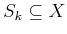must be closed. This means that the cells must all fit together nicely.
2. Each-cell is an open set in the topological subspace. Note that 0-cells are open in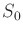, even though they are usually closed in.

Example 6..1 (Vertical Decomposition)   The vertical decomposition of Section 6.2.2 is a nice example of a singular complex that is not a simplicial complex because it contains trapezoids. The interior of each trapezoid and triangle forms a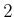-cell, which is an open set. For every pair of adjacent-cells, there is a-cell on their common boundary. There are no 0-cells because the vertices lie in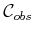, not in. The subspace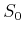is formed by taking the union of all-cells and-cells to yield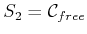. This satisfies the closure requirement because the complex is built inonly; hence, the topological space is. The setis both open and closed. The set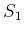is the union of all-cells. This is also closed because the-cell endpoints all lie in. Each-cell is also an open set.

One way to avoid some of these strange conclusions from the topology restricted tois to build the vertical decomposition in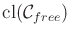, the closure of. This can be obtained by starting with the previously defined vertical decomposition and adding a new-cell for every edge ofand a 0-cell for every vertex of. Now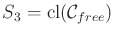, which is closed in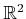. Likewise,,, and, are closed in the usual way. Each of the individual-dimensional cells, however, is open in the topological space. The only strange case is that the 0-cells are considered open, but this is true in the discrete topological space.Steven M LaValle 2020-08-14